# Substring in JavaScript

#### Substring

Substring is an string method in JavaScript, which is used to extract the characters between two specified indices from a string and returns the new substring. It has two parameters 'Start' and 'End'.

In JavaScript, by using `substring()` method we can extract the characters between two specified indices from a string.

EXAMPLE: Extracting characters between position 2 to 5.

```<p>Click the below button to extract.</p>
<input type="button" value="Extract" onclick="Function()" />
<p id="myId"></p>

<script>
function Function() {
var A = "TechFunda";
var r = A.substring(2, 5);
document.getElementById("myId").innerHTML = r;
}
</script>
```

In the above code snippet we have given `Id` as "myId" to the second `<p>` element, there is a string with the variable `A` in the script code. We need to extract the characters between 2, 5 indices in string for that we used the code `.substring(2, 5)`, in this 2 is the start index and 5 is the end index. Onclick of the button "Extract" in the HTML code fires the Function() in the `<script>` code at the same time the string method `substring()` extracts the characters in a string between "start(2)" and "end(5)" indices and returns the output as new substring.

OUTPUT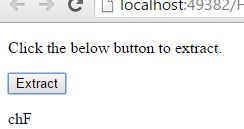NOTE:

• The output includes the character of the 'Start' parameter and does not includes the character of the 'End' parameter.
• Extraction at particular position {Eg: `A.substring(3)`}, extract the rest of the string.
• If strat index is greater than the end index {Eg: `A.substring(5, 2)`}, the `substring()` method will swap the two arguments.
• If start is less than zero(0) {Eg: `A.substring(-2)`}, the `substring()` method extracts from index position zero(0) and returns the new substring as same as the old string.
• If we want to extract only the first character in a string "TechFunda", we need to use the code `A.substring(0, 1)`, it will return only the first character in the new substring.
• If we want to extract only the last character in a string "TechFunda", we need to use the code `A.substring(8, 9)`, it will return only the last character in the new substring.
EXAMPLE: Extracting the whole string from position '3'.
```<p>Click the below button to extract the whole string from position 3.</p>
<input type="button" value="Extract" onclick="Function()" />
<p id="myId"></p>

<script>
function Function() {
var A = "TechFunda";
var r = A.substring(3);
document.getElementById("myId").innerHTML = r;
}
</script>
```
Extracting whole string from position 3

We need to return the whole string from the position 3, for that we are using `substring(3)`. Onclick of the button extracts the whole string from position 3 and returns the extracted part as new substring.

OUTPUT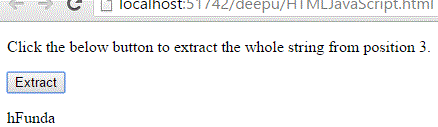EXAMPLE: If Start is greater than End.

```<p>Click the below button to extract.</p>
<input type="button" value="Extract" onclick="Function()" />
<p id="myId"></p>

<script>
function Function() {
var A = "TechFunda";
var r = A.substring(3, 1);
document.getElementById("myId").innerHTML = r;
}
</script>
```

Start is greater than End

In the above code snippet we have given greater value to the 'Start' parameter compared to the value of the 'End' parameter, in this type of cases the `substring()` method swaps two arguments. it includes the 'End' parameter character and does not includes the 'Start' parameter character.

OUTPUT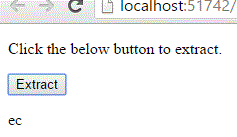EXAMPLE: If 'Start' is negative.

```<p>Click the below button to extract.</p>
<input type="button" value="Extract" onclick="Function()" />
<p id="myId"></p>

<script>
function Function() {
var A = "TechFunda";
var r = A.substring(-1);
document.getElementById("myId").innerHTML = r;
}
</script>
```

In the above code snippet we have given negative value to the `substring()` method, in this type of cases the `substring()` method starts the extraction from '0' and extracts the full string.

OUTPUT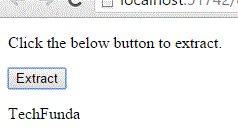EXAMPLE: Extracting the first character.

```<p>Click the below button to extract first character.</p>
<input type="button" value="Extract" onclick="Function()" />
<p id="myId"></p>

<script>
function Function() {
var A = "TechFunda";
var r = A.substring(0, 1);
document.getElementById("myId").innerHTML = r;
}
</script>
```

Extracting first character

We need to extract the first character from a string, for that we are using `substring(0, 1)`. Onclick of the button extracts the first character and returns the extracted character in a new string.

OUTPUT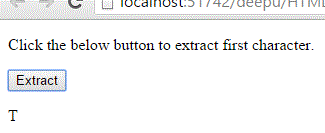EXAMPLE: Extracting last character.

```<p>Click the below button to extract last character.</p>
<input type="button" value="Extract" onclick="Function()" />
<p id="myId"></p>

<script>
function Function() {
var A = "TechFunda";
var r = A.substring(8, 9);
document.getElementById("myId").innerHTML = r;
}
</script>```

Extracting last character

We need to extract the last character from a string, for that we are using `substring(8, 9)`. Onclick of the button extracts the last character and returns the extracted character in a new string.

OUTPUT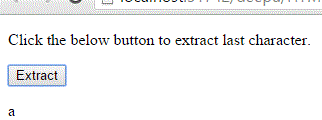Views: 5960 | Post Order: 77